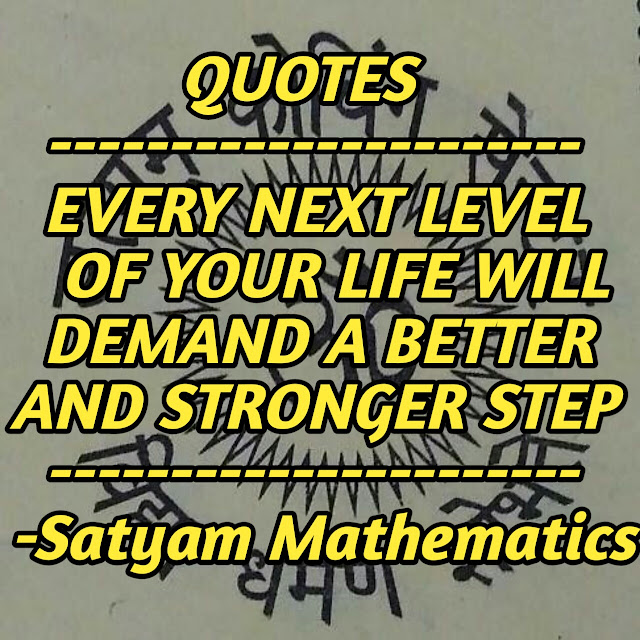Home » » Satyam Mathematics

# Satyam Mathematics

## 1.Mathematics-

The logical study of shape,arrangement,quantity and many related concepts.Mathematics often is divided into three fields:algebra,analysis and geometry,However,no clear divisions can be made,since these branches have become thoroughly intermingled.Roughly,algebra involves numbers and their abstractions,analysis involves continuity and limits and geometry is concerned with space and related concepts.Tech. The postulational science in which necessary conclusion are drawn arc drawn from specified premises.

## 2.Applied mathematics-

A branch of mathematics concered with the study of the physical,biological and sociological worlds.It includes mechanics of rigid and deformable bodies (elasticity,plasticity,mechanics of fluids),theory of electricity and magnetism,relativity,theory of potential,thermodynamics,biomathematics and statistics broadly speaking a mathematical structure utilizing,in addition to the purely mathematical concepts of space and number,the notions of time and matter belongs to the domain of time and matter belongs to the domain of applied mathematics.In a restricted sense,the term refers to the use of mathematical principles as tools in the fields of physics,chemistry,engineering,biology and social studies.

### 3.Mathematics of finance-

The study of the mathematical practices in brokerage,banking and insurance.

### 4.Pure Mathematics-

The study and development of the principles of mathematics for their own sake and possible future usefulness,rather than for their immediate usefulness in other fields of science or knowledge.The study of mathematics independently of experience in other scholarly disciplines.Often the study of problems in applied mathematics leads to new developments in pure mathematics and theories developed as pure mathematics often find applications later.Thus no sharp line can be drawn between applied and pure mathematics.

# We plan to read self everytimeSatyam! Mathematics